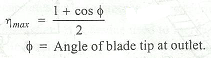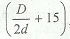# Impulse Turbines

What is an impulse turbine? How do we calculate hydraulic efficiency and mechanical efficiency of an impulse turbine? The following important points may be noted for impulse turbines:

(a) The hydraulic efficiency of an impulse turbine is the ratio of the workdone on the wheel to the energy of the jet.

(b) The hydraulic efficiency of an impulse turbine is maximum when the velocity of wheel is one-half the velocity of jet of water at inlet.

(c) The maximum hydraulic efficiency of an impulse turbine is given by(d) The mechanical efficiency of an impulse turbine is the ratio of the actual work available at the turbine to the energy imparted to the wheel.

(e) The overall efficiency of an impulse turbine is the ratio of the actual power produced by the turbine to the energy actually supplied by the turbine.

(f) The width of the bucket for a Pelton wheel is generally five times the diameter of jet.

(g) The depth of the bucket for a Pelton wheel is generally 1.2 times the diameter of jet.

(h) The number of buckets on the periphery of a Pelton wheel is given by,where D is the pitch diameter of the wheel and d is the diameter of the jet.

(i) The ratio of D / d is called jet ratio.

(j) The maximum number of jets, generally, employed on Pelton wheel are six.